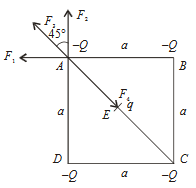#### Four charges equal to   -Q  are placed at the four corners of a square and a charge q   is at its centre If the system is in equilibrium the value of q is  Option 1)Option 2)Option 3)Option 4)As we learnt in

Superposition of Electric field -

The resultant electric field at any point is equal to the vector sum of all the electric fields.

- whereinConsider the four forces F1, F2, F3 and F4  acting on charge (-Q)   placed at AFor equilibrium, consider forces along DA and  equate the resultant to zeroOption 1)Incorrect

Option 2)Correct

Option 3)Incorrect

Option 4)Incorrect

##### Posted by